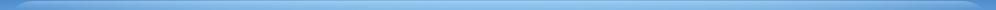学术活动学术会议前沿科学论坛Colloquium专题学术报告/SeminarLunch SeminarCoffee Time研究生高级课程其他学术活动您现在的位置：首页 > 学术活动 > 专题学术报告/Seminar
 (Seminar) Two indices Sachdev-Ye-Kitaev model
 2018-09-12  【大 中 小】【打印】【关闭】
 CAS Key Laboratory of Theoretical Physics Institute of Theoretical Physics Chinese Academy of Sciences Seminar Title 题目 Two indices Sachdev-Ye-Kitaev model Speaker 报告人 Jinwu Ye Affiliation 所在单位 首都师范大学和 Mississippi State University Date 日期 2:30pm, Sep 12, 2018, Wednesday Venue 地点 Conference Room 322, ITP main building Abstract 摘要 We study the original Sachdev-Ye (SY) model in its Majorana fermion representation which can be called the two indices Sachdev-Ye-Kitaev (SYK) model. Its advantage over the original SY model in the $SU(M)$ complex fermion representation is that it need no local constraints, so a $1/M$ expansion can be more easily performed. Its advantage over the 4 indices Sachdev-Ye-Kitaev (SYK) model is that it has only two site indices $J_{ij}$ instead of four indices $J_{ijkl}$, so it may fit the bulk string theory better. By performing a $1/M$ expansion at $N=\infty$, we show that a quantum spin liquid (QSL) state remains stable at a finite $M$. The $1/M$ corrections are exactly marginal, so the system remains conformably invariant at any finite $M$. The 4-point out of time correlation ( OTOC ) shows quantum chaos neither at $N=\infty$ at any finite $M$, nor at $M=\infty$ at any finite $N$. By looking at the replica off-diagonal channel, we find there is a quantum spin glass (QSG) instability at an exponentially suppressed temperature in $M$. We work out a criterion for the two large numbers $N$ and $M$ to satisfy so that the QSG instability may be avoided. We speculate that at any finite $N$, the quantum chaos appears at the order of $1/M^{0}$, which is the subleading order in the $1/M$ expansion. When the $1/N$ quantum fluctuations at any finite $M$ are considered, from a general reparametrization symmetry breaking point of view, we argue that the effective action should still be described by the Schwarzian one, the OTOC shows maximal quantum chaos. We discuss experimental realizations of the two indices SYK. This work may motivate other works to study the possible new gravity dual of the 2 indices SYK model. Contact person 所内联系人 Chushun TianIE6.0浏览器，1024X768分辨率 版权所有 © 中国科学院理论物理研究所 地址：北京市海淀区中关村东路55号　邮政编码：100190【京ICP备05002865号】 京公网安备1101080094号